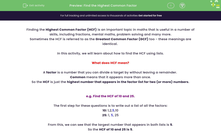# Find the Highest Common Factor

In this worksheet, students will find the Highest Common Factors (HCF) of number pairs by creating lists and finding the highest option which appears in both lists.Key stage:  KS 4

Year:  GCSE

GCSE Subjects:   Maths

GCSE Boards:   Pearson Edexcel, OCR, Eduqas, AQA,

Curriculum topic:   Number, Number Operations and Integers

Curriculum subtopic:   Structure and Calculation Whole Number Theory

Difficulty level:#### Worksheet Overview

Finding the Highest Common Factor (HCF) is an important topic in maths that is useful in a number of skills, including fractions, mental maths, problem solving and many more.

Sometimes the HCF is referred to as the Greatest Common Factor (GCF) too - these meanings are identical.

In this activity, we will learn about how to find the HCF using lists.

What does HCF mean?

A factor is a number that you can divide a target by without leaving a remainder.

Common means that it appears more than once.

So the HCF is just the highest number that appears in the factor list for two (or more) numbers.

e.g. Find the HCF of 10 and 25.

The first step for these questions is to write out a list of all the factors:

10: 1,2,5,10

25: 1, 5, 25

From this, we can see that the largest number that appears in both lists is 5.

So the HCF of 10 and 25 is 5.

In this activity, you will find the HCFs of number pairs by creating lists and finding the highest option which appears in both lists.

You may want to have a pen and paper handy so that you can write out your lists before selecting your answers.

Want a bit more help with this before you begin? Why not watch this short video?

### What is EdPlace?

We're your National Curriculum aligned online education content provider helping each child succeed in English, maths and science from year 1 to GCSE. With an EdPlace account you’ll be able to track and measure progress, helping each child achieve their best. We build confidence and attainment by personalising each child’s learning at a level that suits them.

Get started